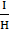# A paramagnetic substance in the form of a cube with sides 1 cm has a magnetic dipole moment of 20 × 10−6 J/T when a magnetic intensity of 60 × 103 A/m is applied. Its magnetic susceptibility is  a. 2.3 × 10−2 b. 3.3 × 10−2 c. 3.3 × 10−4 d. 4.3 × 10−2

## Question ID - 50331 :- A paramagnetic substance in the form of a cube with sides 1 cm has a magnetic dipole moment of 20 × 10−6 J/T when a magnetic intensity of 60 × 103 A/m is applied. Its magnetic susceptibility is  a. 2.3 × 10−2 b. 3.3 × 10−2 c. 3.3 × 10−4 d. 4.3 × 10−2

3537=I =I ==  20 N/m2==×= 0.33 × 103 = 3.3 × 104

Next Question :
 If a, thenis equal to a)b)c)d)